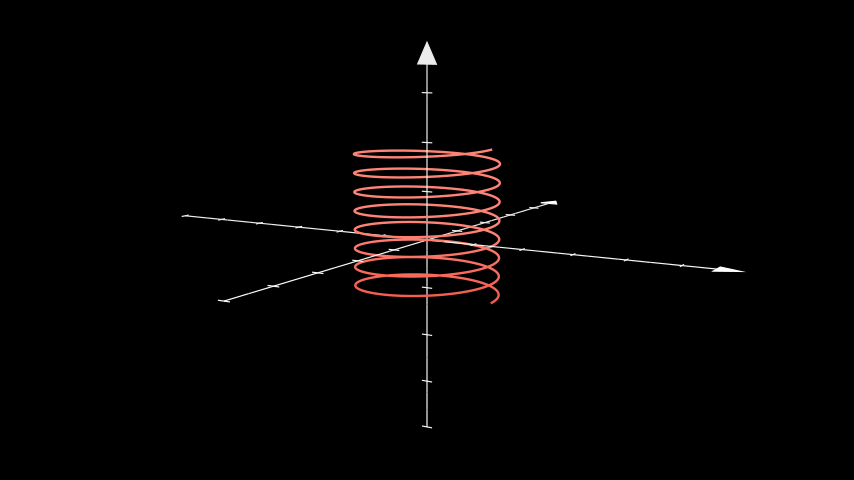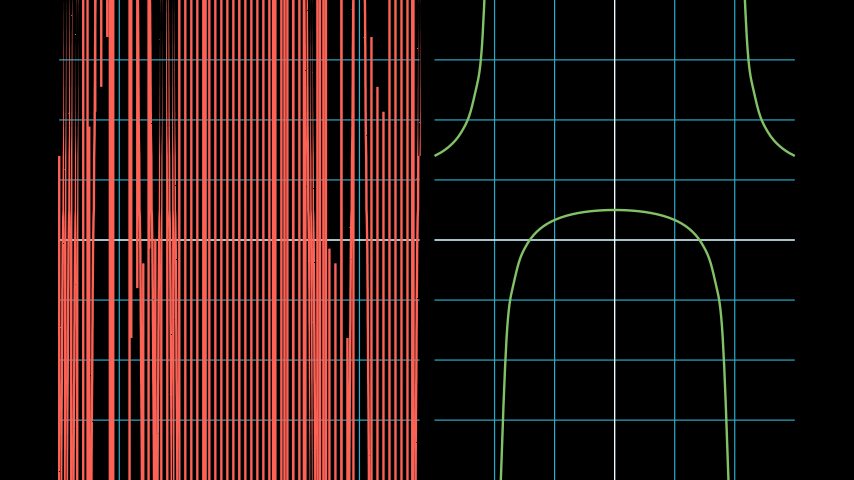# ParametricFunction#

Qualified name: `manim.mobject.graphing.functions.ParametricFunction`

class ParametricFunction(function, t_range=None, scaling=<manim.mobject.graphing.scale.LinearBase object>, dt=1e-08, discontinuities=None, use_smoothing=True, use_vectorized=False, **kwargs)[source]#

Bases: `VMobject`

A parametric curve.

Parameters
• function (Callable[[float, float], float]) – The function to be plotted in the form of `(lambda x: x**2)`

• t_range (Sequence[float] | None) – Determines the length that the function spans. By default `[0, 1]`

• scaling (_ScaleBase) – Scaling class applied to the points of the function. Default of `LinearBase`.

• use_smoothing (bool) – Whether to interpolate between the points of the function after they have been created. (Will have odd behaviour with a low number of points)

• use_vectorized (bool) – Whether to pass in the generated t value array to the function as `[t_0, t_1, ...]`. Only use this if your function supports it. Output should be a numpy array of shape `[[x_0, x_1, ...], [y_0, y_1, ...], [z_0, z_1, ...]]` but `z` can also be 0 if the Axes is 2D

• discontinuities (Iterable[float] | None) – Values of t at which the function experiences discontinuity.

• dt (float) – The left and right tolerance for the discontinuities.

Examples

Example: PlotParametricFunction```from manim import *

class PlotParametricFunction(Scene):
def func(self, t):
return np.array((np.sin(2 * t), np.sin(3 * t), 0))

def construct(self):
func = ParametricFunction(self.func, t_range = np.array([0, TAU]), fill_opacity=0).set_color(RED)
```

Example: ThreeDParametricSpring```from manim import *

class ThreeDParametricSpring(ThreeDScene):
def construct(self):
curve1 = ParametricFunction(
lambda u: np.array([
1.2 * np.cos(u),
1.2 * np.sin(u),
u * 0.05
]), color=RED, t_range = np.array([-3*TAU, 5*TAU, 0.01])
axes = ThreeDAxes()
self.set_camera_orientation(phi=80 * DEGREES, theta=-60 * DEGREES)
self.wait()
```

Attention

If your function has discontinuities, you’ll have to specify the location of the discontinuities manually. See the following example for guidance.

Example: DiscontinuousExample```from manim import *

class DiscontinuousExample(Scene):
def construct(self):
ax1 = NumberPlane((-3, 3), (-4, 4))
ax2 = NumberPlane((-3, 3), (-4, 4))
VGroup(ax1, ax2).arrange()
discontinuous_function = lambda x: (x ** 2 - 2) / (x ** 2 - 4)
incorrect = ax1.plot(discontinuous_function, color=RED)
correct = ax2.plot(
discontinuous_function,
discontinuities=[-2, 2],  # discontinuous points
dt=0.1,  # left and right tolerance of discontinuity
color=GREEN,
)
```

Methods

 `generate_points` Initializes `points` and therefore the shape. `get_function` `get_point_from_function` `init_points` Initializes `points` and therefore the shape.

Attributes

 `animate` Used to animate the application of any method of `self`. `animation_overrides` `color` `depth` The depth of the mobject. `fill_color` If there are multiple colors (for gradient) this returns the first one `height` The height of the mobject. `n_points_per_curve` `sheen_factor` `stroke_color` `width` The width of the mobject.
generate_points()[source]#

Initializes `points` and therefore the shape.

Gets called upon creation. This is an empty method that can be implemented by subclasses.

init_points()#

Initializes `points` and therefore the shape.

Gets called upon creation. This is an empty method that can be implemented by subclasses.